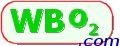Oxygen Percentage & Lambda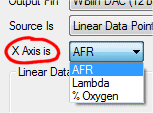This page discusses the relationship between Lambda (or AFR) and percentage oxygen as measured by a wideband sensor connected to a Tech Edge controller.

Tech Edge supplies a utility to re-program the voltage (and current) outputs of its controllers. It's possible to directly specify the output in terms of percent oxygen.

Wideband sensors measure both excess oxygen (in a lean environment) and also oxygen deficit (ie. how much oxygen is required for complete combustion in an incompletely combusted gas mixture). How they do this is described here.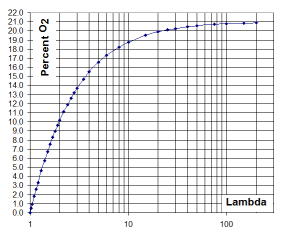Percent O2 & Lambda (λ) Conversion

For their sensors, Bosch have described a formula for calculating lambda (λ) from percent oxygen (xO2) - it only works correctly in the lean region where % oxygen is positive, and it also assumes the H/C (Hydrogen to Carbon) ratio is 2.0. Here K is a constant and, depending on the Bosch document, is either 4.76 or 4.77 (it depends on what you define as the free-air oxygen concentration). Also xO2 is a fraction, eg. 0.209 for free-air:

• xO2 = 3 * (λ - 1) / (1 + 3 * K * λ)

• λ = (xO2 / 3 + 1) / (1 - K * xO2)

We have graphed the relationship and it's shown at right using lambda as the X-axis (log scale), & percent oxygen as the Y-axis.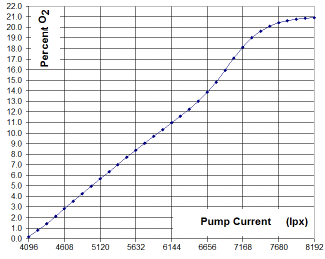Percent O2 and Pump Current (Ip)

Percentage oxygen is a direct measure of how many oxygen molecules there are in the gas sample. As described here, a wideband sensor has a small gas measuring chamber (diffusion chamber) that is supplied with a small pump current to "neutralise" the excess oxygen molecules (when there is an excess of oxygen, it is transported, in the form of oxygen ions, out of the diffusion chamber to free-air). In this way, the pump current is a direct measure of percentage oxygen in the sampled gas.

On Tech Edge controllers, rather than Lambda, the primary measure from the sensor is Ipx (normalised pump current*) which represents the oxygen-ion current inside the sensor. Bosch documentation refers to Ip, and our Ipx is a normalised version of Ip where a value of 4096 represents zero pump current, and 8192 is the value achieved in free-air (which is assumed to be 20.9% oxygen). As complex chemical processes occur inside the sensor, a pump current of zero is close to, but not exactly, zero percent oxygen.

We have graphed the relationship between Ipx and % oxygen as shown at right using Ipx as the X-axis (linear scale), & percent oxygen as the Y-axis. Note that this is a relatively "linear" relationship. In an ideal sensor and controller the curve may be more linear but the relationship is more complex than a direct straight line.

*Note: Ipx has an operational range between 0 and 8191 although it can be larger than 8191 and remains valid. The process of free-air calibration discovers a constant (called the cal-value, γ) which. when applied to Ip (raw pump current), produces the normalise Ipx value.

• Ipx = f(γ) * Ip

Ideal vs. Real-World Gas measurements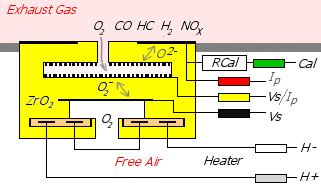It is important to recognise potential sources of error when using wideband oxygen sensors. Here are some reminders:

• Wideband sensors work equally well in rich and lean (ie. where there's an excess of fuel or an excess of oxygen). The practical implication of this is that a mixture of unburnt gaseous fuel + oxygen can give a zero % result. In some applications this may be a problem, and in others a necessity. Importantly, the sensor is not actually measuring absolute oxygen concentration but the balance between hydrocarbon fuels and oxygen.

• Vaporised liquid fuels (or aerosols) will not be detected in the same way that gaseous fuels will be. For example, misfires (ie. fuel does not ignite) in internal combustion (IC) engines running vapourised fuels (petrol/unleaded) will be seen as a lean condition because the exhaust vapour (very small fuel droplets) will not enter the sensor's diffusion chamber (but the oxygen will). On the other hand, if the fuel is LPG (propane + butane, in the form of a gas in the exhaust), the sensor will not detect there has been a misfire.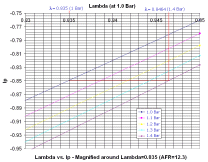• The sensor's working parts are heated to a dull red heat by an integrated heater with a maximum power of about 9 Watts. The controller cannot maintain the sensor's working temperature if the external gas has a large enough convective cooling capacity (ie. high speed and/or cool gas). The sensor can only be heated by the controller, not cooled. There are protection tubes covering the sensor element that have the ability to cool exhaust gas hotter than the sensor's heater. Measurement errors will result if the sensor is forced outside its working temperature. Most controllers have an LED indication to warn when the sensor is operating outside its temperature range.

• All oxygen sensors are sensitive to their working pressure. Chemical reactions within the sensor primarily depend on the partial pressure of oxygen. As the pressure in a gas increases, its density (ie. number of gas molecules per unit volume) increases. If a sensor's measuring chamber has more gas molecules, then more electrons (ie. a larger electric current) is needed to measure the same gas concentration. This is discussed further here.

Wideband Sensors: Application Limitations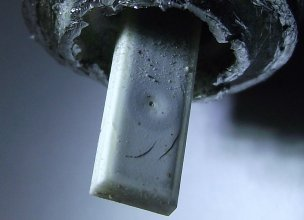Wideband sensors are primarily used in automotive applications where certain assumptions are made about how they are used. People normally think of automotive exhausts as fairly dirty but the reality is that modern engines, whether running on unleaded, LPG or diesel, are relatively clean. In fact, for vehicles with factory-fitted wideband oxygen sensors, most of the time the vehicle will be running at its cleanest and most efficient operating point meaning the sensor will rarely experience adverse operating conditions.

Many non-automotive applications will use wideband sensors in a flue or as part of a combustion process. In these situations there may be many sources of physical and chemical contamination. We have a page devoted to broken and contaminated sensors which we update regularly.

Another problem is that sensors can fail mechanically if they are subjected to thermal stress from water condensing inside the sensor (water is a combustion by-product, usually in the form of steam). There are mounting and usage considerations we have outlined here.